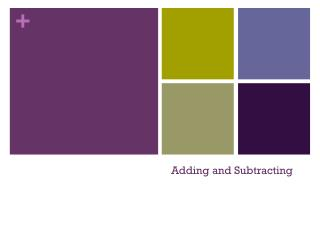DownloadDownload PresentationTélécharger la présentation- - - - - - - - - - - - - - - - - - - - - - - - - - - E N D - - - - - - - - - - - - - - - - - - - - - - - - - - -
##### Presentation Transcript

2. How do you add or subtract fractions?

3. Adding and Subtracting Rational Expressions: • The addition and subtraction of fractions can be carried out only when their denominators are identical. • To get a required denominator, we may build up the denominator of a fraction. • We multiply the numerator and denominator of a fraction by the same number (not zero) to get an equivalent fraction. Definition: Addition and Subtraction of Rational Numbers If a/b and c/b are rational numbers, then and

4. Example:

5. Adding and Subtracting with Unlike Denominators • For fractions with different denominators, we build up one or both denominators to make the denominators the equal. • The least common denominator (LCD) is the smallest number that is a multiple of all of the denominators. • Procedure: Finding the LCD • Factor each denominator completely. • Write a product using each factor that appears in a denominator. • For each factor, use the highest power of that factor that occurs in the denominators.

6. Example: Combine the fractions: Solution:

7. Example: Combine the fractions: Solution:

8. Example: Combine the fractions: Solution:

9. Example: Combine the fractions: Solution:

10. Assignment